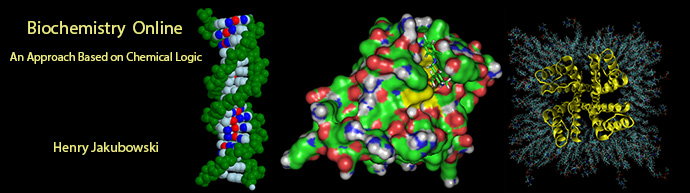# Biochemistry Online: An Approach Based on Chemical Logic# CHAPTER 5 - BINDING

## B:  MATHEMATICAL ANALYES OF BINDING GRAPHS

Last Updated: 3/25/16

 Learning Goals/Objectives for Chapter 5B:  After class and this reading, students will be able to derive equations  from the equation for fractional saturation  Y = ([ML]/[M0] = [L]/(KD+ [L])  equations for 1/Y as a function of 1/[L] (double reciprocal plot), Y/[L] vs Y (bound/free vs bound or the Scatchard Plot) and draw a plot of Y vs log [L] (semilog plot) draw error bars on the plots of Y vs L, 1/Y vs I/L, Y/L vs Y and Y vs log L. explain from these derivative equations and graphs how to calculate determine Kd describe appropriate methods to fit the data from these equations with special attention given to the effect of error bars in the experimental value of YNavigation

Archived version of full Chapter 5B:  Mathematical Analyses of Binding Graphs